Next: Reduction Theorems Up: Complexity Previous: Xia's Reduction Algorithm

## Late-breaking News ...

Close to the deadline for this article the author was mailed the preprint , in which it is proved that problem 2.3 is indeed NP-complete. One of the main obstacles to such a proof is the large number of possibilities for order-preserving maps on an ordered set, which makes a reduction to 3SAT difficult. For example the sets used in the second proof of Theorem 2.14 are all dismantlable (cf. Example 3.9, part 1) to a 2-8m complete bipartite ordered set and thus do not have the fixed point property. In fact they have many fixed point free order-preserving maps, while the possible fixed point free automorphisms are restricted by many constraints (which is of course what makes the argument work). In  Duffus and Goddard present a beautiful class of ordered sets that is such that if a member has a fixed point free order-preserving map, then it must have a very ``well-behaved" order-preserving map. This nice structure then allows to embed another NP-complete problem into ordered sets.
The ordered sets used in  are quite special, so interesting questions regarding the complexity of the fixed point property remain, as for example the questions in  and questions 1 and 6 in section 5.7 indicate. Regarding complexity of the fixed point property in general, one might now be interested how difficult problem 2.3 is ``on average" (since NP-completeness is a worst case concept). For an introduction to average case complexity  cf. J. Wang's survey . Also the sets in  can be used to construct ordered sets with the fixed point property for which Xia's reduction algorithm terminates with a graph that still has edges: In fact, the set constructed from the non-satisfiable, 3-variable, 8-clause instance of 3SAT has by  the fixed point property and it was proved (in collaboration with M. Roddy) that Xia's algorithm terminates with a graph that has edges left. The ordered set in question has 434 points and its fixed-point-free endomorphism graph has in excess of 80,000 vertices. Presently no smaller sets with this property are known. Again, for the proof that problem 2.3 is NP-complete the reader is referred to , which is self-contained and nothing short of a complete reproduction could do it complete justice here.
Xia's ideas are a more versatile tool than indicated here:   If P,Q are finite sets (with possible additional structures), then for any set of functions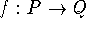that for all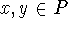satisfy conditions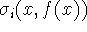and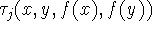(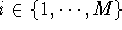,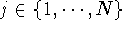,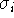,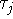logical propositions) we can construct the Xia graph as follows: The vertices are all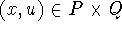such that all conditions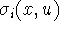are satisfied and the edges are all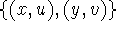such that all conditions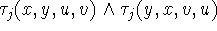are satisfied. Then as in Theorem 2.7 we have a natural correspondence between these maps and the maximum sized cliques in the Xia graph. Xia's algorithm can be used and if it terminates with a graph with no edges, there is no map satisfying all conditions. If Xia leaves some edges, a subsequent depth-first search can settle the question of existence, resp. determine the number of maps. Thus Xia's algorithm can be used as a (for existence problems highly efficient) preprocessor when confronting problems such as: Enumeration and existence of relation preserving self maps with special properties, the isomorphism problem for graphs, determination of rigidity of graphs or ordered sets, existence of fixed point free automorphisms, etc. All that is needed is a formulation of all the properties of the maps as indicated above.
The magnitude of the improvement Xia provides in various settings is currently under investigation (). It appears that using Xia's algorithm (plus depth-first search if necessary) the fixed point property for ordered sets with up to 100 points can be decided on a desktop PC with enough RAM.Next: Reduction Theorems Up: Complexity Previous: Xia's Reduction Algorithm

Bernd.S.W.Schroder